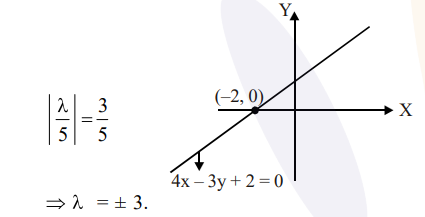# Lines are drawn parallel to the line 4 x-3 y+2=0,`
Question:

Lines are drawn parallel to the line $4 x-3 y+2=0$,

at a distance $\frac{3}{5}$ from the origin.

Then which one of the following points lies on any of these lines?

1. $\left(-\frac{1}{4}, \frac{2}{3}\right)$

2. $\left(\frac{1}{4}, \frac{1}{3}\right)$

3. $\left(-\frac{1}{4},-\frac{2}{3}\right)$

4. $\left(\frac{1}{4},-\frac{1}{3}\right)$

Correct Option: 1

Solution:

Required line is $4 x-3 y+\lambda=0$So, required equation of line is $4 x-3 y+3=0$ and $4 x-3 y-3=0$

(1) $4\left(-\frac{1}{4}\right)-3\left(\frac{2}{3}\right)+3=0$